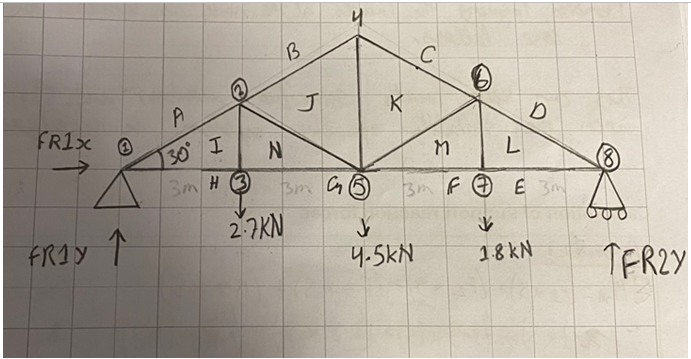# What I have done is draw a space diagram for the given system. A space diagram is a diagram drawn to a scale. I have used a scale of 1 square = 1 meter. I have also added forces that act on this system.

Complete checks to find the support reactions, and establish that the framework is stable and statically determinate. Also, prepare using a suitable scale and annotation of a space diagram for the framework.

Space diagram of the system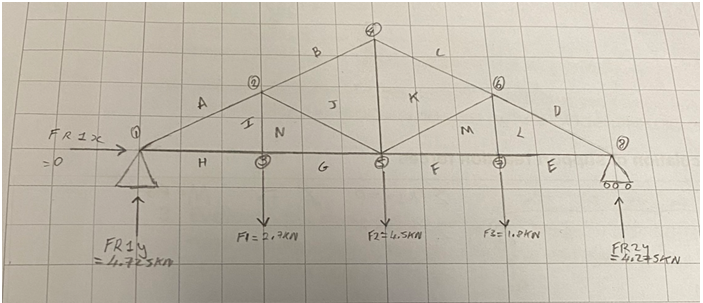What I have done is draw a space diagram for the given system. A space diagram is a diagram drawn to a scale. I have used a scale of 1 square = 1 meter. I have also added forces that act on this system.

I have also labeled each node using numbers from left to right, so they can be referred to. A node is where two or more members are connected together via a pin. I also labeled each space adjoining the members using upper case letters. The sequence of letters goes clockwise from outside to inside creating a spiral. Lastly, I have included the supported beams.

Node table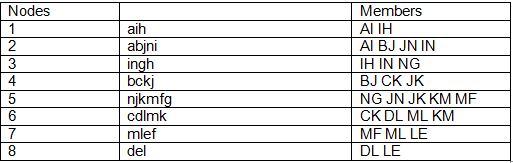The spaces adjoining a node are labeled as lowercase letters.

Members forming the joints at the node are labeled as upper case letters.

This can help locate specific members and joints when the stability of the system is analyzed.

Calculation of support reaction forces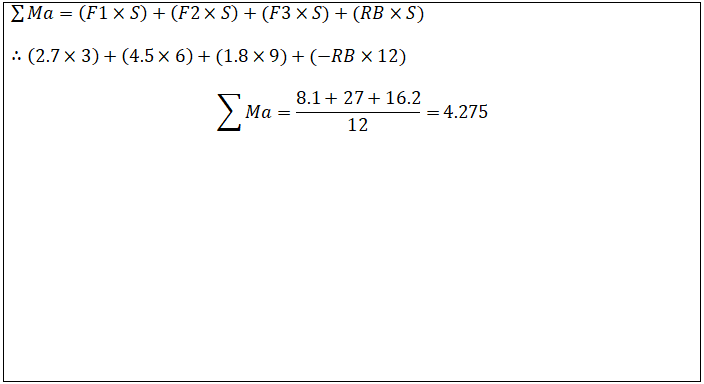Stability and static determinacy

There should be enough reaction force to balance the system, for example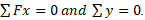To check the stability of the structure I have carried out multiple checks to see if the system is stable firstly I have carried out calculations to show that the forces in the system are balanced. Then I made sure that the reaction forces in the system are not all parallel with each other because in this diagram one end is supported by a pin and the other by a roller. Also, there is two reaction forces that are vertical and one is horizontal

This framework is stable because the system is entirely made up of triangles which prevent the structure from internally collapsing.

To establish internal static determinacy?

Internal static determinacy is where all external reactions and internal reactions may be calculated using static equilibrium alone.